## Monday, 21 December 2015

### Simplifying Expressions and Solving Equations Tower Challenge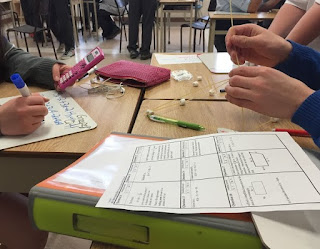This is a review activity on simplifying expressions and solving equations for grade 9 applied where students answer questions and are rewarded with building materials for each correct answer. The building materials (spagetti & marshmallows) are then used with the goal being the creation of tallest tower. This is based originally on a TIPS activity on quadratics for MBF3C (Unit 3, Day 6).  We have a similar activity for grade 9 academic that can be found here.

MPM 1P
• substitute into and evaluate algebraic expressions involving exponents
• describe the relationship between the algebraic and geometric representations of a single-variable term up to degree three [i.e., length, which is one dimensional, can be represented by x; area, which is two dimensional, can be represented by (x)(x) or x2; volume, which is three dimensional,can be represented by (x)(x)(x), (x2)(x),or x3]
• add and subtract polynomials involving the same variable up to degree three using a variety of tools
• multiply a polynomial by a monomial involving the same variable to give results up to degree three using a variety of tools
• solve first-degree equations with nonfractional coefficients, using a variety of tools
• substitute into algebraic equations and solve for one variable in the first degree
• 1 bag of spaghetti and 1-2 bags of small marshmallows (or 1 box of straws and 1-inch pieces of tape)
• a question sheet for each student
• Optional - a whiteboard for each student to work out their solutions
• Optional - prize for the group with the tallest tower1. Place students in groups (ideally no bigger than 3 per group)
2. Hand out question sheets (and optional whiteboards) to each student.
3. Have students answer questions from their sheet in any order they want. For every correct answer they will get some building materials (eg: 2 spagetti & 3 marshmallows, the amount of each reward is indicated on the student question sheet ). The harder the question the more materials they will get. Eventually the building materials will be used to create a tower with the goal to create the tallest free standing tower.
4. Students work in groups to answer the questions and bring their solutions up to you to be checked. Only one member from each group can come up at a time. Each group can answer each question only once. To keep track of this, use the teacher answer sheet to check off which questions each group has answered as they come up.
5. Leave about 20 min at the end of the class for students to create their towers (students can no longer answer questions)
6. Take lots of pictures and celebrate the group with the tallest free standing tower.

• Gr9AppliedSimplifyingExpression&SolvingEquationsTowerChallengeQuestions (pdfdoc)
Did you use this activity? Do you have a way to make it better? If so tell us in the comment section. Thanks

## Sunday, 20 December 2015

### Solving Equations Balance Method Card Sort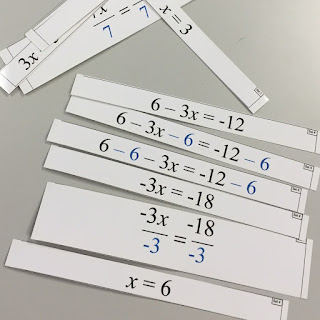This is a simple activity where students are given a set of cards that represent the steps to take the given equation and use the balance method to solve it. The equations are relatively simple with most being two step solutions. This is not meant to be a big activity but just a warm up or perhaps something to do in the middle of class to break it up. There are 9 equations that can be solved so you could put students in groups or make multiple sets and have them work on them individually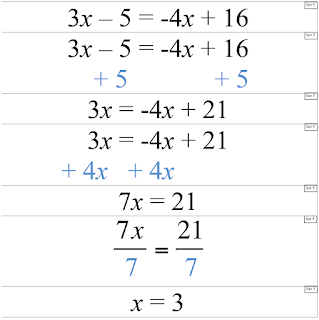• There are 9 sets of cards to print out and cut. We suggest printing on card stock and laminating. To help, each card will have it's set number just in case you mix up the cards. If you wish to use this with individual students rather than in groups you will need to print out more than one full set.
• NOTE - there are two versions. One with the traditional adding and subtracting of terms on the same line and the other with the adding and subtracting of terms done below. Obviously choose the version you prefer. The equations are the same in both
• We suggest putting each set in it's own envelop or zip-lock bag.

1. Randomly distribute the envelopes to groups (or individual students).
2. Have students use all the cards to show the steps to solve their question
3. Students should check their answer on paper or portable whiteboard
4. Once all groups (students) have correctly sorted their cards, they can exchange their set with the next group (since the sets are numbered they can just get the next numbered set - if they have set 1 then pass it to the group with set 2 etc).
5. This could be done 9 times or stopped when ever you wish.

• Solving Equations Card Sort Horizontal (pdf, doc)
• Solving Equations Card Sort Vertical (pdf, doc)

Did you use this activity? Do you have a way to make it better? If so tell us in the comment section. Thanks

## Friday, 18 December 2015

### Number Sense and Algebra Tower ChallengeThis is a review activity on number sense and algebra for grade 9 academic where students answer questions and are rewarded with building materials for each correct answer. The building materials (spagetti & marshmallows) are then used with the goal being the creation of tallest tower. This is based originally on a TIPS activity on quadratics for MBF3C (Unit 3, Day 6).  We have a similar activity for grade 9 applied that can be found here

MPM 1D
• substitute into and evaluate algebraic expressions involving exponents
• describe the relationship between the algebraic and geometric representations of a single-variable term up to degree three [i.e., length, which is one dimensional, can be represented by x; area, which is two dimensional, can be represented by (x)(x) or x2; volume, which is three dimensional,can be represented by (x)(x)(x), (x2)(x),or x3]
• derive, through the investigation and examination of patterns, the exponent rules for multiplying and dividing monomials, and apply these rules in expressions involving one and two variables with positive exponents
• extend the multiplication rule to derive and understand the power of a power rule, and apply it to simplify expressions involving one and two variables with positive exponents.
• relate their understanding of inverse operations to squaring and taking the square root, and apply inverse operations to simplify expressions and solve equations
• add and subtract polynomials with up to two variables
• multiply a polynomial by a monomial involving the same variable
• expand and simplify polynomial expressions involving one variable
• solve first-degree equations, including equations with fractional coefficients, using a variety of tools
• rearrange formulas involving variables in the first degree, with and without substitution
• solve problems that can be modelled with first-degree equations, and compare algebraic methods to other solution methods
• 1 bag of spaghetti and 1-2 bags of small marshmallows (or 1 box of straws and 1-inch pieces of tape)
• a question sheet for each student
• Optional - a whiteboard for each student to work out their solutions
• Optional - prize for the group with the tallest tower1. Place students in groups (ideally no bigger than 3 per group)
2. Hand out question sheets (and optional whiteboards) to each student.
3. Have students answer questions from their sheet in any order they want. For every correct answer they will get some building materials (eg: 2 spagetti & 3 marshmallows, the amount of each reward is indicated on the student question sheet ). The harder the question the more materials they will get. Eventually the building materials will be used to create a tower with the goal to create the tallest free standing tower.
4. Students work in groups to answer the questions and bring their solutions up to you to be checked. Only one member from each group can come up at a time. Each group can answer each question only once. To keep track of this, use the teacher answer sheet to check off which questions each group has answered as they come up.
5. Leave about 20 min at the end of the class for students to create their towers (students can no longer answer questions)
6. Take lots of pictures and celebrate the group with the tallest free standing tower

Did you use this activity? Do you have a way to make it better? If so tell us in the comment section. Thanks

### I Have, Who Has - Equations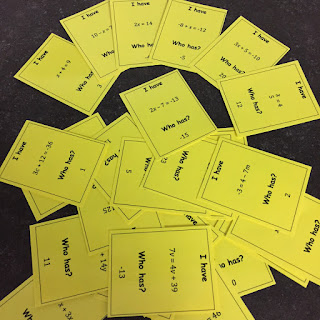An I Have, Who Has game is not a new concept. The premiss is that each person gets a card that has two statements. One is the "I have" statement and the other is the "Who has" statement. In this case the "I have" statement is a simple equation "Who has" statement which is the answer. The way the game works is that a person starts by reading their "Who has" statement. For example, someone might say "Who has 7?". Someone else will have a card where their equation has an answer that equals 7 so they would say " I have 2x = 14". Who has negative 4?" That is, they read their equation that has an answer that equals 7 and then asks their "Who has" statement. Then someone else will have an equation that matches -4 and the game continues. If done correctly, it should end up with the person who started giving their "I have" statement. It works really well as a warm up and one of nice things about this is that you could do it multiple days and kids will likely get different cards.

• Gr7PA - solve linear equations of the form ax = c or c = ax and ax + b = c or variations such as b + ax = c and c = bx + a (where a, b, and c are natural numbers) by modelling with concrete materials, by inspection, or by guess and check, with and without the aid of a calculator NOTE - only use the 9 card set if doing this for grade 7 as there are a few multi step equations;
• Gr8PA - solve and verify linear equations involving a one-variable term and having solutions that are integers, by using inspection, guess and check, and a “balance” model
• MPM1D, MFM1P - solve first-degree equations, including equations with fractional coefficients, using a variety of tools (e.g., computer algebra systems, paper and pencil) and strategies (e.g., the balance analogy, algebraic strategies);• There are two sets of cards that you could download here. One set (pictured here) has only 9 cards in it (you can see that the card on the top left has the "I have" to match the "Who has" of the card on the bottom right). Depending on the size of class you have you might want to use this set multiple times (ie groups of 9) or use the larger set of 27. Either way, in order for the game to work, all cards need to be passed out. So some students may need to have more than one card.
• Regardless. Print out the set you want (ideally on coloured card stock) and we also suggest lamination to lengthen the lifespan of the cards.
• Be sure to print out a set for yourself that you don't cut out so that it will be easier for you to check as students play the game.
1. Distribute the cards one per student. All cards must be handed out so some students might need more than one card.
2. Tell each person to solve their "I have" equation and check their answer with at least one other person.
3. Once students are confident with their answer all students should stand and then you choose one to read their "Who has" statement. The person who's equation has the same answer should read their "I have" statement followed by their "Who has" statement and then sit down. Eventually the last person standing should be the person who started.
4. A variation might be to have students walk to the front and stand next to the person who they were matched with and eventually form an entire loop around the class.
• IHaveWhoHas-Equations-9cards (pdf) (doc)
• IHaveWhoHas-Equationss-27cards (pdf) (doc)
• IHaveWhoHas-BlankTemplate (doc)
Did you use this activity? Do you have a way to make it better? If so tell us in the comment section. Thanks

## Wednesday, 16 December 2015

### Simplifying Expressions with Algebra Tiles Matching CardsThis is a very simple matching activity where students are given a bunch of algebraic expressions (in one variable) and simplified expressions (both in algebraic and algebra tile form) and all they have to do is match up each expression with their simplified form. This is not meant to be a long or complex activity and could best be used as a warm up or end of class review or possibly just in the middle of a long class to switch up the period.
• MPM1D - add and subtract polynomials with up to two variables [e.g., (2x – 5) + (3x + 1), (3x2y + 2xy2) + (4x2y – 6xy2)], using a variety of tools (e.g., algebra tiles, computer algebra systems, paper and pencil);
• MFM1P - add and subtract polynomials involving the same variable up to degree three [e.g., (2x + 1) + (x2– 3x + 4)], using a variety of tools (e.g., algebra tiles, computer algebra systems, paper and pencil);• There are four sets of cards (each group would get a set so they aren't all working on the same questions). Each set of cards will have answers that have the same characteristics (Set 1 - answer is a trinomial, Set 2 - answer is a binomial with no squared term, Set 3 answer is a binomial with no x term, Set 4 - answer is a binomial with no constant term). A set should contain 12 cards. Four long expressions, four algebraic answers and four algebra tile answers.
• Print and cut out the cards (we suggest laminating them to). Note that each card has what set number it belongs to so that if you mix them up you can easily sort them back into their sets. You may wish to put each set in an envelope or ziplock bag for easy distribution.
• You will probably want to have physical algebra tiles for students to use while doing the actual simplification.
• Note that because there doesn't seem to be a consensus on which colour algebra tiles represent positive or negative, there are two sets. One that assumes red is positive and one that assumes red is negative. Keep which ever is the protocol for your class and discard the other.

1. Students could be put into groups or do this individually. Ether way, since there are only four sets you will need to have multiple copies of each set.
2. Give each group an envelop and tiles and ask them to match the unsimplified expression with the simplified expression and algebra tile representation.
3. Once each group is done, have groups switch their envelopes. (1 gives to 2, 2 gives to 3 etc). You can do this 4 times.

• SimplifyingPolynomialsMatching (pdf, doc)
Did you use this activity? Do you have a way to make it better? If so tell us in the comment section. Thanks

## Monday, 14 December 2015

### I Have, Who Has - Proportions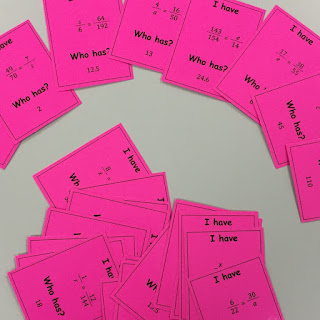An I Have, Who Has game is not a new concept. The premiss is that each person gets a card that has two statements. One is the "I have" statement and the other is the "Who has" statement. In this case the "I have" statement is an proportion with a missing value and a "Who has" statement which is the answer. The way the game works is that a person starts by reading their "Who has" statement. For example, someone might say "Who has 24?". Someone else will have a card where their proportion has an answer that equals 24 so they would say " I have 8 over x equals 5 over 15". Who has 56?" That is, they read their proportion that has an answer that equals 24 and then asks their "Who has" statement. Then someone else will have an expression that matches 56 and the game continues. If done correctly, it should end up with the person who started giving their "I have" statement. It works really well as a warm up and one of nice things about this is that you could do it multiple days and kids will likely get different cards.

• Gr8PA - solve and verify linear equations involving a one-variable term and having solutions that are integers, by using inspection, guess and check, and a “balance” model NOTE - only use the 9 card set if doing this for grade 8;
• MPM1D - solve problems requiring the manipulation of expressions arising from applications of percent, ratio, rate, and proportion
• MPM1D, MFM1P - solve first-degree equations, including equations with fractional coefficients, using a variety of tools (e.g., computer algebra systems, paper and pencil) and strategies (e.g., the balance analogy, algebraic strategies);
• MFM1P - solve for the unknown value in a proportion, using a variety of methods• There are two sets of cards that you could download here. One set (pictured here) has only 9 cards in it (you can see that the card on the top left has the "I have" to match the "Who has" of the card on the bottom right). Depending on the size of class you have you might want to use this set multiple times (ie groups of 9) or use the larger set of 27. Either way, in order for the game to work, all cards need to be passed out. So some students may need to have more than one card.
• Regardless. Print out the set you want (ideally on coloured card stock) and we also suggest lamination to lengthen the lifespan of the cards.
• Be sure to print out a set for yourself that you don't cut out so that it will be easier for you to check as students play the game.
1. Distribute the cards one per student. All cards must be handed out so some students might need more than one card.
2. Tell each person to answer their "I have" proportion and check their answer with at least one other person.
3. Once students are confident with their answer all students should stand and then you choose one to read their "Who has" statement. The person who's answer is the same should read their "I have" statement followed by their "Who has" statement and then sit down. Eventually the last person standing should be the person who started.
4. A variation might be to have students walk to the front and stand next to the person who they were matched with and eventually form an entire loop around the class.
• IHaveWhoHas-Proportions-9cards (pdf) (doc)
• IHaveWhoHas-Proportions-27cards (pdf) (doc)
• IHaveWhoHas-BlankTemplate (doc)
Did you use this activity? Do you have a way to make it better? If so tell us in the comment section. Thanks

### I Have, Who Has - Angles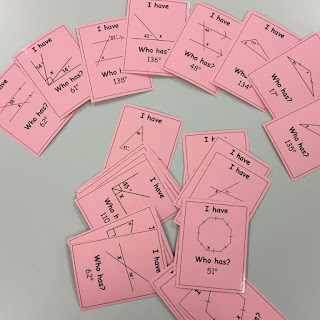An I Have, Who Has game is not a new concept. The premiss is that each person gets a card that has two statements. One is the "I have" statement and the other is the "Who has" statement. In this case the "I have" statement is an diagram dealing with determining an angle and a "Who has" statement which is the angle. The way the game works is that a person starts by reading their "Who has" statement. For example, someone might say "Who has 138o?". Someone else will have a card where their diagram has an angle that equals 138o so they would say " I have x = 138o". Who has 136o?" That is, they read their angle that equals 138o and then asks their "Who has" statement. Then someone else will have an expression that matches 136o and the game continues. If done correctly, it should end up with the person who started giving their "I have" statement. It works really well as a warm up and one of nice things about this is that you could do it multiple days and kids will likely get different cards.
In this set there are questions for parallel lines with a transversal, complimentary angles, supplementary angles, exterior angles, sum of interior angles and opposite angles questions

• Gr8GSS - solve angle-relationship problems involving triangles (e.g., finding interior angles
or complementary angles), intersecting lines (e.g., finding supplementary angles or opposite angles), and parallel lines and transversals (e.g., finding alternate angles or corresponding angles) NOTE - only use the 9 card set if doing this for grade 8;
• MPM1D - determine, through investigation using a variety of tools (e.g., dynamic geometry software, paper folding), and describe some properties of polygons, and apply the results in problem solving
• MPM1D, MFM1P - determine, through investigation using a variety of tools (e.g., dynamic geometry software, concrete materials), and describe the properties and relationships of the interior and exterior angles of triangles, quadrilaterals, and other polygons, and apply the results to problems involving the angles of polygons
• MFM1P - determine, through investigation using a variety of tools (e.g., dynamic geometry software, concrete materials), and describe the properties and relationships of the angles formed by parallel lines cut by a transversal, and apply the results to problems involving parallel lines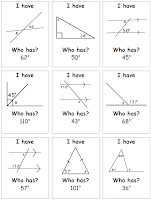• There are two sets of cards that you could download here. One set (pictured here) has only 9 cards in it (you can see that the card on the top left has the "I have" to match the "Who has" of the card on the bottom right). Depending on the size of class you have you might want to use this set multiple times (ie groups of 9) or use the larger set of 27. Either way, in order for the game to work, all cards need to be passed out. So some students may need to have more than one card.
• Regardless. Print out the set you want (ideally on coloured card stock) and we also suggest lamination to lengthen the lifespan of the cards.
• Be sure to print out a set for yourself that you don't cut out so that it will be easier for you to check as students play the game.
1. Distribute the cards one per student. All cards must be handed out so some students might need more than one card.
2. Tell each person to determine the indicated angle in their "I have" diagram and check their answer with at least one other person.
3. Once students are confident with their answer all students should stand and then you choose one to read their "Who has" statement. The person who's answer is the same should read their "I have" statement followed by their "Who has" statement and then sit down. Eventually the last person standing should be the person who started.
4. A variation might be to have students walk to the front and stand next to the person who they were matched with and eventually form an entire loop around the class.
• IHaveWhoHas-Angles-9cards (pdf) (doc)
• IHaveWhoHas-Angles-27cards (pdf) (doc)
• IHaveWhoHas-BlankTemplate (doc)
Did you use this activity? Do you have a way to make it better? If so tell us in the comment section. Thanks